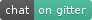Pingouin is an open-source statistical package written in Python 3 and based mostly on Pandas and NumPy. Some of its main features are listed below. For a full list of available functions, please refer to the API documentation.

1. ANOVAs: N-ways, repeated measures, mixed, ancova

2. Pairwise post-hocs tests (parametric and non-parametric) and pairwise correlations

3. Robust, partial, distance and repeated measures correlations

4. Linear/logistic regression and mediation analysis

5. Bayes Factors

6. Multivariate tests

7. Reliability and consistency

8. Effect sizes and power analysis

9. Parametric/bootstrapped confidence intervals around an effect size or a correlation coefficient

10. Circular statistics

11. Chi-squared tests

12. Plotting: Bland-Altman plot, Q-Q plot, paired plot, robust correlation…

Pingouin is designed for users who want simple yet exhaustive stats functions.

For example, the ttest_ind function of SciPy returns only the T-value and the p-value. By contrast, the ttest function of Pingouin returns the T-value, the p-value, the degrees of freedom, the effect size (Cohen’s d), the 95% confidence intervals of the difference in means, the statistical power and the Bayes Factor (BF10) of the test.

# Installation

Pingouin is a Python 3 package and is currently tested for Python 3.6 and 3.7. Pingouin does not work with Python 2.7.

The main dependencies of Pingouin are :

• NumPy (>= 1.15)

• SciPy (>= 1.3.0)

• Pandas (>= 0.24)

• Pandas-flavor (>= 0.1.2)

• Matplotlib (>= 3.0.2)

• Seaborn (>= 0.9.0)

In addition, some functions require :

• Statsmodels

• Scikit-learn

• Mpmath

Pingouin can be easily installed using pip

pip install pingouin


or conda

conda install -c conda-forge pingouin


Pingouin is under heavy development and it is likely that bugs/mistakes will be discovered in future releases. Please always make sure that you are using the latest version of Pingouin (new releases are frequent, i.e. about one per month).

To be notified whenever a new release of Pingouin is available, you can click on “Watch releases” on the GitHub of Pingouin (see below).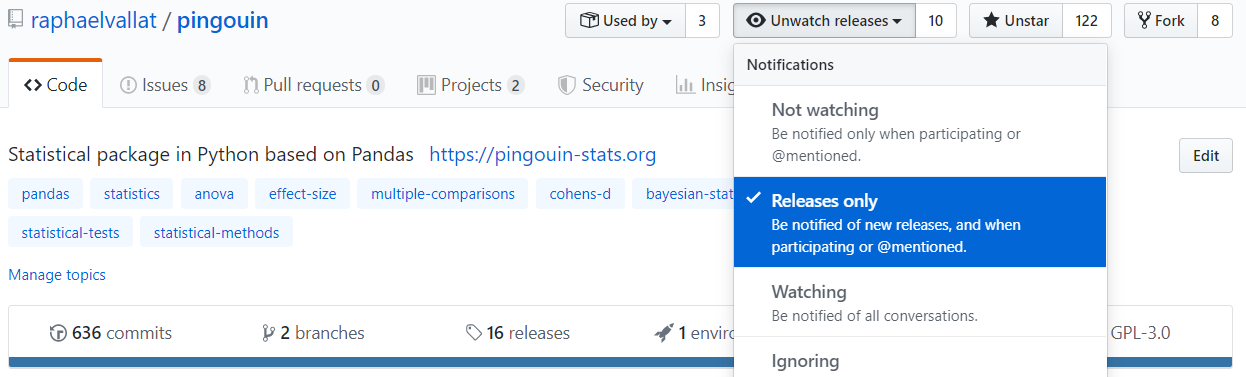Whenever a new release is out there, you can upgrade your version by typing the following line in a terminal window:

pip install --upgrade pingouin


# Quick start

## 10 minutes to Pingouin

### 1. T-test

import numpy as np
import pingouin as pg

np.random.seed(123)
mean, cov, n = [4, 5], [(1, .6), (.6, 1)], 30
x, y = np.random.multivariate_normal(mean, cov, n).T

# T-test
pg.ttest(x, y)

Output

T

dof

tail

p-val

CI95%

cohen-d

BF10

power

-3.401

58

two-sided

0.001

[-1.68 -0.43]

0.878

26.155

0.917

### 2. Pearson’s correlation

pg.corr(x, y)

Output

n

r

CI95%

r2

p-val

BF10

power

30

0.595

[0.3 0.79]

0.354

0.306

0.001

69.723

0.95

### 3. Robust correlation

# Introduce an outlier
x = 18
# Use the robust Shepherd's pi correlation
pg.corr(x, y, method="shepherd")

Output

n

r

CI95%

r2

p-val

power

30

0.561

[0.25 0.77]

0.315

0.264

0.002

0.917

### 4. Test the normality of the data

The pingouin.normality() function works with lists, arrays, or pandas DataFrame in wide or long-format.

print(pg.normality(x))                                    # Univariate normality
print(pg.multivariate_normality(np.column_stack((x, y)))) # Multivariate normality

Output

W

pval

normal

0.615

0.000

False

(False, 0.00018)


### 5. Q-Q plot

import numpy as np
import pingouin as pg
np.random.seed(123)
x = np.random.normal(size=50)
ax = pg.qqplot(x, dist='norm')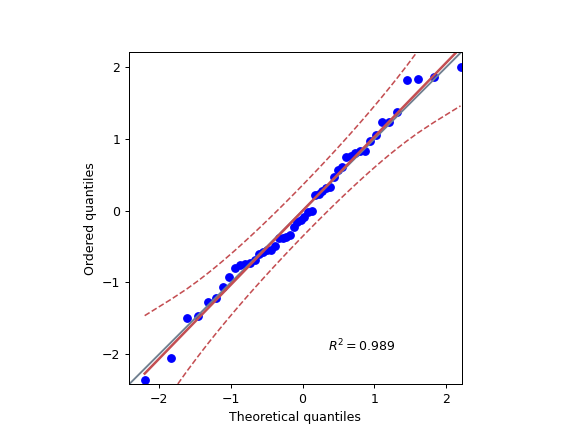### 6. One-way ANOVA using a pandas DataFrame

# Read an example dataset

# Run the ANOVA
aov = pg.anova(data=df, dv='Scores', between='Group', detailed=True)
print(aov)

Output

Source

SS

DF

MS

F

p-unc

np2

Group

5.460

1

5.460

5.244

0.02320

0.029

Within

185.343

178

1.041

### 7. Repeated measures ANOVA

pg.rm_anova(data=df, dv='Scores', within='Time', subject='Subject', detailed=True)

Output

Source

SS

DF

MS

F

p-unc

np2

eps

Time

7.628

2

3.814

3.913

0.022629

0.062

0.999

Error

115.027

118

0.975

### 8. Post-hoc tests corrected for multiple-comparisons

# FDR-corrected post hocs with Hedges'g effect size
posthoc = pg.pairwise_ttests(data=df, dv='Scores', within='Time', subject='Subject',

# Pretty printing of table
pg.print_table(posthoc, floatfmt='.3f')

Output

Contrast

A

B

Paired

Parametric

T

dof

tail

p-unc

p-corr

BF10

CLES

hedges

Time

August

January

True

True

-1.740

59.000

two-sided

0.087

0.131

fdr_bh

0.582

0.585

-0.328

Time

August

June

True

True

-2.743

59.000

two-sided

0.008

0.024

fdr_bh

4.232

0.644

-0.485

Time

January

June

True

True

-1.024

59.000

two-sided

0.310

0.310

fdr_bh

0.232

0.571

-0.170

### 9. Two-way mixed ANOVA

# Compute the two-way mixed ANOVA and export to a .csv file
aov = pg.mixed_anova(data=df, dv='Scores', between='Group', within='Time',
subject='Subject', correction=False,
export_filename='mixed_anova.csv')
pg.print_table(aov)

Output

Source

SS

DF1

DF2

MS

F

p-unc

np2

eps

Group

5.460

1

58

5.460

5.052

0.028

0.080

Time

7.628

2

116

3.814

4.027

0.020

0.065

0.999

Interaction

5.168

2

116

2.584

2.728

0.070

0.045

### 10. Pairwise correlations between columns of a dataframe

import pandas as pd
np.random.seed(123)
z = np.random.normal(5, 1, 30)
data = pd.DataFrame({'X': x, 'Y': y, 'Z': z})
pg.pairwise_corr(data, columns=['X', 'Y', 'Z'])

Output

X

Y

method

tail

n

r

CI95%

r2

z

p-unc

BF10

power

X

Y

pearson

two-sided

30

0.366

[0.01 0.64]

0.134

0.070

0.384

0.047

1.500

0.525

X

Z

pearson

two-sided

30

0.251

[-0.12 0.56]

0.063

-0.006

0.256

0.181

0.534

0.272

Y

Z

pearson

two-sided

30

0.020

[-0.34 0.38]

0.000

-0.074

0.020

0.916

0.228

0.051

### 11. Convert between effect sizes

# Convert from Cohen's d to Hedges' g
pg.convert_effsize(0.4, 'cohen', 'hedges', nx=10, ny=12)

0.384


### 12. Multiple linear regression

pg.linear_regression(data[['X', 'Z']], data['Y'])

Linear regression summary

names

coef

se

T

pval

r2

CI[2.5%]

CI[97.5%]

Intercept

4.650

0.841

5.530

0.000

0.139

0.076

2.925

6.376

X

0.143

0.068

2.089

0.046

0.139

0.076

0.003

0.283

Z

-0.069

0.167

-0.416

0.681

0.139

0.076

-0.412

0.273

### 13. Mediation analysis

pg.mediation_analysis(data=data, x='X', m='Z', y='Y', seed=42, n_boot=1000)

Mediation summary

path

coef

se

pval

CI[2.5%]

CI[97.5%]

sig

Z ~ X

0.103

0.075

0.181

-0.051

0.256

No

Y ~ Z

0.018

0.171

0.916

-0.332

0.369

No

Total

0.136

0.065

0.047

0.002

0.269

Yes

Direct

0.143

0.068

0.046

0.003

0.283

Yes

Indirect

-0.007

0.025

0.898

-0.070

0.029

No

### 14. Contingency analysis

data = pg.read_dataset('chi2_independence')
expected, observed, stats = pg.chi2_independence(data, x='sex', y='target')
stats

Chi-squared tests summary

test

lambda

chi2

dof

p

cramer

power

pearson

1.000

22.717

1.000

0.000

0.274

0.997

0.667

22.931

1.000

0.000

0.275

0.998

log-likelihood

0.000

23.557

1.000

0.000

0.279

0.998

freeman-tukey

-0.500

24.220

1.000

0.000

0.283

0.998

mod-log-likelihood

-1.000

25.071

1.000

0.000

0.288

0.999

neyman

-2.000

27.458

1.000

0.000

0.301

0.999

### 15. Bland-Altman plot

import numpy as np
import pingouin as pg
np.random.seed(123)
mean, cov = [10, 11], [[1, 0.8], [0.8, 1]]
x, y = np.random.multivariate_normal(mean, cov, 30).T
ax = pg.plot_blandaltman(x, y)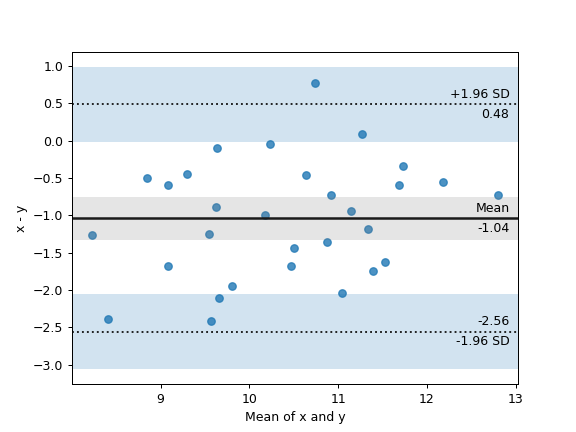### 16. Plot achieved power of a paired T-test

Plot the curve of achieved power given the effect size (Cohen d) and the sample size of a paired T-test.

import matplotlib.pyplot as plt
import seaborn as sns
import pingouin as pg
import numpy as np
sns.set(style='ticks', context='notebook', font_scale=1.2)
d = 0.5  # Fixed effect size
n = np.arange(5, 80, 5)  # Incrementing sample size
# Compute the achieved power
pwr = pg.power_ttest(d=d, n=n, contrast='paired', tail='two-sided')
# Start the plot
plt.plot(n, pwr, 'ko-.')
plt.axhline(0.8, color='r', ls=':')
plt.xlabel('Sample size')
plt.ylabel('Power (1 - type II error)')
plt.title('Achieved power of a paired T-test')
sns.despine()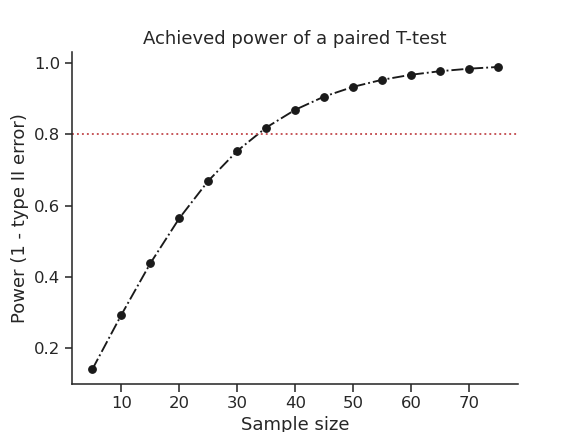### 17. Paired plot

import pingouin as pg
import numpy as np
df = pg.read_dataset('mixed_anova').query("Group == 'Meditation' and Time != 'January'")
ax = pg.plot_paired(data=df, dv='Scores', within='Time', subject='Subject', dpi=150)
ax.set_title("Effect of meditation on school performance")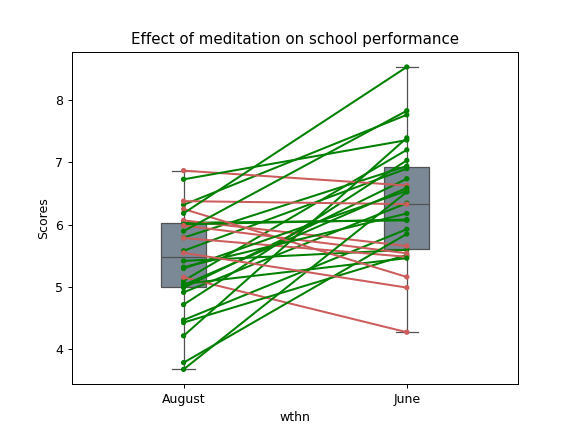## Integration with Pandas

Several functions of Pingouin can be used directly as pandas.DataFrame methods. Try for yourself with the code below:

import pingouin as pg

# Example 1 | ANOVA
df.anova(dv='Scores', between='Group', detailed=True)

# Example 2 | Pairwise correlations
data.pairwise_corr(columns=['X', 'M', 'Y'], covar=['Mbin'])

# Example 3 | Partial correlation matrix
data.pcorr()


The functions that are currently supported as pandas method are:

# Development

Pingouin was created and is maintained by Raphael Vallat, mostly during his spare time. Contributions are more than welcome so feel free to contact me, open an issue or submit a pull request!

To see the code or report a bug, please visit the GitHub repository.

Note that this program is provided with NO WARRANTY OF ANY KIND. If you can, always double check the results with another statistical software.

# How to cite Pingouin?

If you want to cite Pingouin, please use the publication in JOSS:

Vallat, R. (2018). Pingouin: statistics in Python. Journal of Open Source Software, 3(31), 1026, https://doi.org/10.21105/joss.01026

@ARTICLE{Vallat2018,
title    = "Pingouin: statistics in Python",
author   = "Vallat, Raphael",
journal  = "The Journal of Open Source Software",
volume   =  3,
number   =  31,
pages    = "1026",
month    =  nov,
year     =  2018
}


# Acknowledgement

Several functions of Pingouin were inspired from R or Matlab toolboxes, including:

I am also grateful to Charles Zaiontz and his website www.real-statistics.com which has been useful to understand the practical implementation of several functions.